Last update: 18.10.2021

# 1. Overview

How to measure fairness of a machine learning model?

To date, a number of algorithmic fairness metrics have been proposed. Demographic parity, proportional parity and equalized odds are among the most commonly used metrics to evaluate fairness across sensitive groups in binary classification problems. Multiple other metrics have been proposed based on performance measures extracted from the confusion matrix (e.g., false positive rate parity, false negative rate parity).

Together with Tibor V. Varga, we developed `fairness` package for R. The package provides tools to calculate fairness metrics across different sensitive groups. It also provides opportunities to visualize and compare other prediction metrics between the groups.

This blog post provides a tutorial on using the `fairness` package on a COMPAS data set. The package is published on CRAN and GitHub.

The package implements the following fairness metrics:

• Demographic parity (also known as independence)
• Proportional parity
• Equalized odds (also known as separation)
• Predictive rate parity
• False positive rate parity
• False negative rate parity
• Accuracy parity
• Negative predictive value parity
• Specificity parity
• ROC AUC parity
• MCC parity

# 2. Installation

The latest stable version published on CRAN is `fairness 1.2.2` (as of 14.04.2021). You can install this from CRAN by running:

```install.packages('fairness')
library(fairness)
```
```Installing package into ‘/usr/local/lib/R/site-library’
(as ‘lib’ is unspecified)

```

You may also install the development version from Github to get the latest features:

```devtools::install_github('kozodoi/fairness')
library(fairness)
```

# 3. Data description

The package includes two exemplary data sets to study fairness: `compas` and `germancredit`.

## compas

This tutorial uses a simplified version of the landmark COMPAS data set containing the criminal history of defendants from Broward County. You can read more about the data here. To load the data, all you need to do is:

```data('compas')
```
Two_yr_Recidivism Number_of_Priors Age_Above_FourtyFive Age_Below_TwentyFive Female Misdemeanor ethnicity probability predicted
1 no -0.68435 no no Male yes Other 0.31515 0
2 yes 2.26688 no no Male no Caucasian 0.88546 1
3 no -0.68435 no no Female yes Caucasian 0.25526 0
4 no -0.68435 no no Male no African_American 0.41739 0
5 no -0.68435 no no Male yes Hispanic 0.32009 0

The data set contains nine variables. The outcome variable is `Two_yr_Recidivism`, a binary indicator showing whether an individual committed a crime within the two-year period. The data also includes features on prior criminal record (`Number_of_Priors`, `Misdemeanor`), features describing age (`Age_Above_FourtyFive`, `Age_Below_TwentyFive`), sex and ethnicity (`Female`, `ethnicity`).

For illustrative purposes, we have already trained a classifier that uses all features to predict `Two_yr_Recidivism` and concatenated the predicted probabilities (`probability`) and predicted classes (`predicted`) to the data frame. Feel free to use these columns with predictions to test different fairness metrics before evaluating a custom model.

## germancredit

The second included data set is a credit scoring data set labeled as `germancredit`. The data includes 20 features describing the loan applicants and a binary outcome variable `BAD` indicating whether the applicant defaulted on a loan. Similarly to COMPAS, `germancredit` also includes two columns with model predictions named `probability` and `predicted`. The data can be loaded with:

```data('germancredit')
```

# 4. Train a classifier

For the purpose of this tutorial, we will train two classifiers using different sets of features:

• model that uses all features as input
• model that uses all features except for ethnicity

We partition the COMPAS data into training and validation subsets and use logistic regression as a base classifier.

```#collapse-show

# extract data
compas <- fairness::compas
df     <- compas[, !(colnames(compas) %in% c('probability', 'predicted'))]

# partitioning params
set.seed(77)
val_percent <- 0.3
val_idx     <- sample(1:nrow(df))[1:round(nrow(df) * val_percent)]

# partition the data
df_train <- df[-val_idx, ]
df_valid <- df[ val_idx, ]

# check dim
print(nrow(df_train))
print(nrow(df_valid))
```
``` 4320
 1852
```

```#collapse-show

# fit logit models
model1 <- glm(Two_yr_Recidivism ~ .,
data   = df_train,
model2 <- glm(Two_yr_Recidivism ~ . -ethnicity,
data   = df_train,
```

Let's append model predictions to the validation set. Later, we will evaluate fairness of the two models based on these predictions.

```#collapse-show

# produce predictions
df_valid\$prob_1 <- predict(model1, df_valid, type = 'response')
df_valid\$prob_2 <- predict(model2, df_valid, type = 'response')
```
Two_yr_Recidivism Number_of_Priors Age_Above_FourtyFive Age_Below_TwentyFive Female Misdemeanor ethnicity prob_1 prob_2
1 no -0.68435 no no Male no African_American 0.36787 0.34815
2 no 2.05607 no no Male no Hispanic 0.80241 0.83477
3 yes -0.47355 no yes Male no African_American 0.58958 0.57305
4 no -0.68435 yes no Male no African_American 0.23956 0.22189
5 yes 0.58045 no no Male no Caucasian 0.59155 0.60107

# 5. Intro to algorithimc fairness

## An outlook on the confusion matrix

Most fairness metrics are calculated based on a confusion matrix produced by a classification model. The confusion matrix is comprised of four classes:

• True positives (TP): the true class is positive and the prediction is positive (correct classification)
• False positives (FP): the true class is negative and the prediction is positive (incorrect classification)
• True negatives (TN): the true class is negative and the prediction is negative (correct classification)
• False negatives (FN): the true class is positive and the prediction is negative (incorrect classification)

Fairness metrics are calculated by comparing one or more of these measures across sensitive subgroups (e.g., male and female). For a detailed overview of measures coming from the confusion matrix and precise definitions, click here or here.

## Fairness metrics functions

The package implements 11 fairness metrics. Many of these are mutually exclusive: results for a given classification problem often cannot be fair in terms of all metrics. Depending on a context, it is important to select an appropriate metric to evaluate fairness.

Below, we describe functions used to compute the implemented metrics. Every function has a similar set of arguments:

• `data`: data.frame containing the input data and model predictions
• `group`: column name indicating the sensitive group (factor variable)
• `base`: base level of the sensitive group for fairness metrics calculation
• `outcome`: column name indicating the binary outcome variable
• `outcome_base`: base level of the outcome variable (i.e., negative class) for fairness metrics calculation

We also need to supply model predictions. Depending on the metric, we need to provide either probabilistic predictions as `probs` or class predictions as `preds`. The model predictions can be appended to the original data.frame or provided as a vector. In this tutorial, we will use probabilistic predictions with all functions. When working with probabilistic predictions, some metrics require a cutoff value to convert probabilities into class predictions supplied as `cutoff`.

The package also supports a continuous `group` variable (e.g., age). If `group` is continuous, a user need to supply `group_breaks` argument to specify breaks in the variable values. More details are provided in the functions documentation.

Before looking at different metrics, we will create a binary numeric version of the outcome variable that we will supply as `outcome` in fairness metrics functions. We can also work with an original factor outcome `Two_yr_Recidivism` but in this case we should make sure that predictions and outcome have the same factor levels.

```df_valid\$Two_yr_Recidivism_01 <- ifelse(df_valid\$Two_yr_Recidivism == 'yes', 1, 0)
```

# 6. Computing fairness metrics

## Predictive rate parity

Let's demonstrate the fairness pipeline using predictive rate parity as an example. Predictive rate parity is achieved if the precisions (or positive predictive values) in the subgroups are close to each other. The precision stands for the number of the true positives divided by the total number of examples predicted positive within a group.

Formula: TP / (TP + FP)

Let's compute predictive rate parity for the first model that uses all features:

```res1 <- pred_rate_parity(data         = df_valid,
outcome      = 'Two_yr_Recidivism_01',
outcome_base = '0',
group        = 'ethnicity',
probs        = 'prob_1',
cutoff       = 0.5,
base         = 'Caucasian')
res1\$Metric
```
Caucasian African_American Asian Hispanic Native_American Other
Precision 0.585034 0.702381 0.5000000 0.5909091 1.000000 0.900000
Predictive Rate Parity 1.000000 1.200581 0.8546512 1.0100423 1.709302 1.538372
Group size 622 962 160 1440 4 104

The first row shows the raw precision values for all ethnicities. The second row displays the relative precisions compared to Caucasian defendants.

In a perfect world, all predictive rate parities should be equal to one, which would mean that precision in every group is the same as in the base group. In practice, values are going to be different. The parity above one indicates that precision in this group is relatively higher, whereas a lower parity implies a lower precision. Observing a large variance in parities should hint us that the model is not performing equally well for different groups.

If the other ethnic group is set as a base group (e.g. Hispanic), the raw precision values do not change, only the relative metrics:

```res1h <- pred_rate_parity(data         = df_valid,
outcome      = 'Two_yr_Recidivism_01',
outcome_base = '0',
group        = 'ethnicity',
probs        = 'prob_1',
cutoff       = 0.5,
base         = 'Hispanic')
res1h\$Metric
```
Hispanic Caucasian African_American Asian Native_American Other
Precision 0.5909091 0.5850340 0.702381 0.5000000 1.000000 0.900000
Predictive Rate Parity 1.0000000 0.9900576 1.188645 0.8461538 1.692308 1.523077
Group size 1440 6220 962 160 4 104

Overall, results suggest that the model precision varies between 0.5 and 1. The lowest precision is observed for Asian defendants. This implies that there are more cases where the model mistakingly predicts that a person will commit a crime among Asians than among, e.g., Native_American defendants.

A standard output of every fairness metric function includes a barchart that visualizes the relative metrics for all subgroups:

```res1h\$Metric_plot
```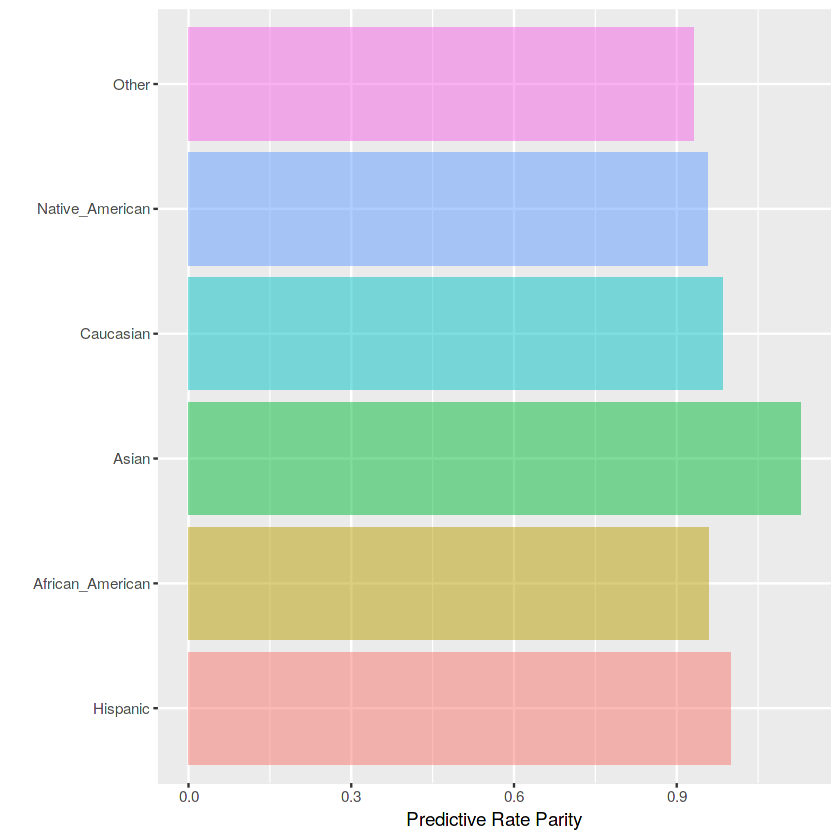Some fairness metrics do not require probabilistic predictions and can work with class predictions. When predicted probabilities are supplied, the output includes a density plot displaying the distributions of probabilities in all subgroups:

```res1h\$Probability_plot
```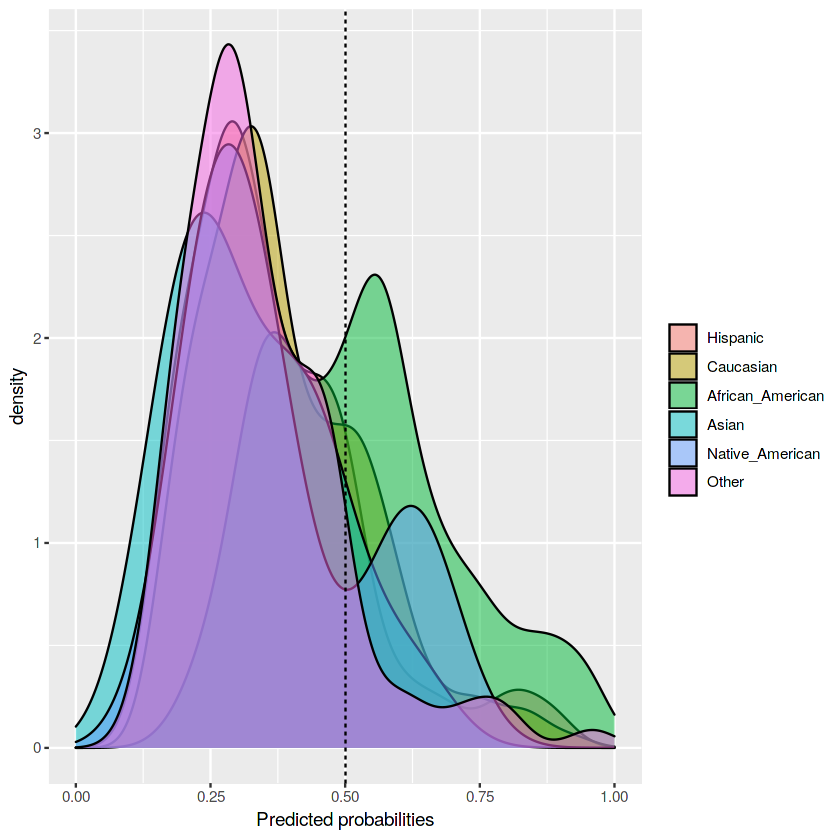Let's now compare the results to the second model that does not use ethnicity as a feature:

```# model 2
res2 <- pred_rate_parity(data         = df_valid,
outcome      = 'Two_yr_Recidivism_01',
outcome_base = '0',
group        = 'ethnicity',
probs        = 'prob_2',
cutoff       = 0.5,
base         = 'Caucasian')
res2\$Metric
```
Caucasian African_American Asian Hispanic Native_American Other
Precision 0.5933333 0.703854 0.4000000 0.5142857 1.000000 0.625000
Predictive Rate Parity 1.0000000 1.186271 0.6741573 0.8667737 1.685393 1.053371
Group size 6220 962 160 1440 4 104

We can see two things.

First, excluding `ethnicity` from the features slightly increases precision for some defendants (Caucasian and African_American) but results in a lower precision for some other groups (Asian and Hispanic). This illustrates that improving a model for one group may cost a fall in the predictive performance for the general population. Depending on the context, it is a task of a decision-maker to decide what is best.

Second, excluding `ethnicity` does not align the predictive rate parities substantially closer to one. This illustrates another important research finding: removing a sensitive variable does not guarantee that a model stops discriminating. Ethnicity correlates with other features and is still implicitly included in the input data. In order to make the classifier more fair, one would need to consider more sophisticated techniques than simply dropping the sensitive attribute.

In the rest of this tutorial, we will go through the functions that cover the remaining implemented fairness metrics, illustrating the corresponding equations and outputs. You can find more details on each of the fairness metric functions in the package documentation. Please don't hesitate to use the built-in helper to see further details and examples on the implemented metrics:

```?fairness::pred_rate_parity
```

## Demographic parity

Demographic parity is one of the most popular fairness indicators in the literature. Demographic parity is achieved if the absolute number of positive predictions in the subgroups are close to each other. This measure does not take true class into consideration and only depends on the model predictions. In some literature, demographic parity is also referred to as statistical parity or independence.

Formula: (TP + FP)

```res_dem <- dem_parity(data         = df_valid,
outcome      = 'Two_yr_Recidivism_01',
outcome_base = '0',
group        = 'ethnicity',
probs        = 'prob_1',
cutoff       = 0.5,
base         = 'Caucasian')
res_dem\$Metric
```
Caucasian African_American Asian Hispanic Native_American Other
Positively classified 147 504 2.00000000 22.0000000 1.000000000 10.00000000
Demographic Parity 1 3.428571 0.01360544 0.1496599 0.006802721 0.06802721
Group size 622 962 1600 1440 4000 10400
```res_dem\$Metric_plot
```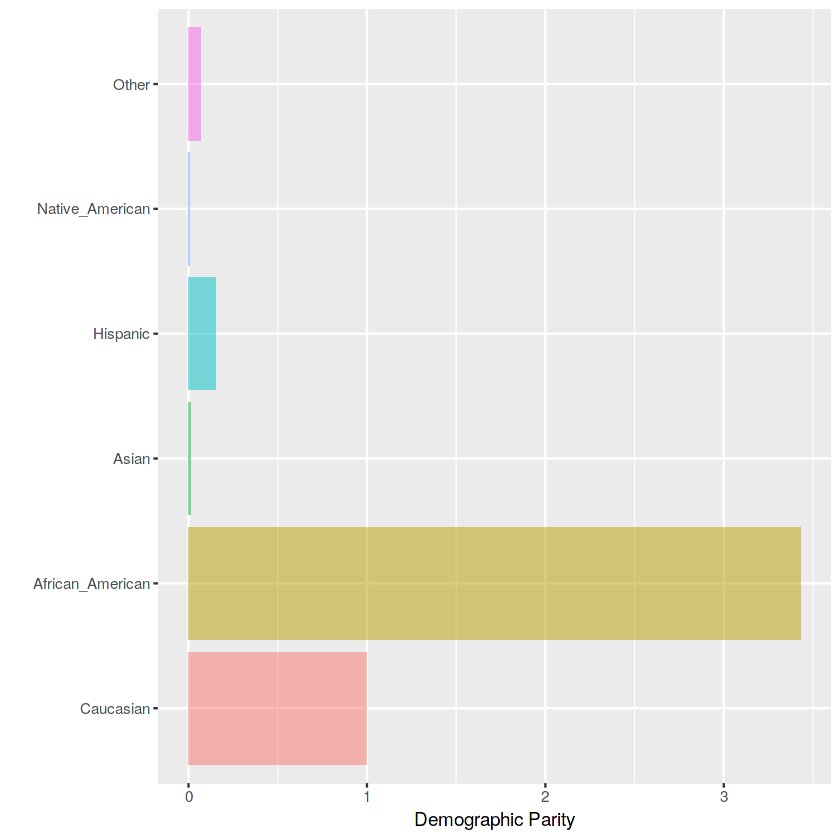Of course, comparing the absolute number of positive predictions will show a high disparity when the number of cases within each group is different, which artificially boosts the disparity. This is true in our case:

```table(df_valid\$ethnicity)
```
```       Caucasian African_American            Asian         Hispanic
622              962               16              144
Native_American            Other
4              104 ```

To address this, we can use proportional parity.

## Proportional parity

Proportional parity is very similar to demographic parity but modifies it to address the issue discussed above. Proportional parity is achieved if the proportion of positive predictions in the subgroups are close to each other. Similar to the demographic parity, this measure also does not depend on the true labels.

Formula: (TP + FP) / (TP + FP + TN + FN)

```res_prop <- prop_parity(data         = df_valid,
outcome      = 'Two_yr_Recidivism_01',
outcome_base = '0',
group        = 'ethnicity',
probs        = 'prob_1',
cutoff       = 0.5,
base         = 'Caucasian')
res_prop\$Metric
```
Caucasian African_American Asian Hispanic Native_American Other
Proportion 0.2363344 0.5239085 0.1250000 0.1527778 0.250000 0.09615385
Proportional Parity 1.0000000 2.2168102 0.5289116 0.6464475 1.057823 0.40685505
Group size 6220 962 160 1440 4 10400
```res_prop\$Metric_plot
```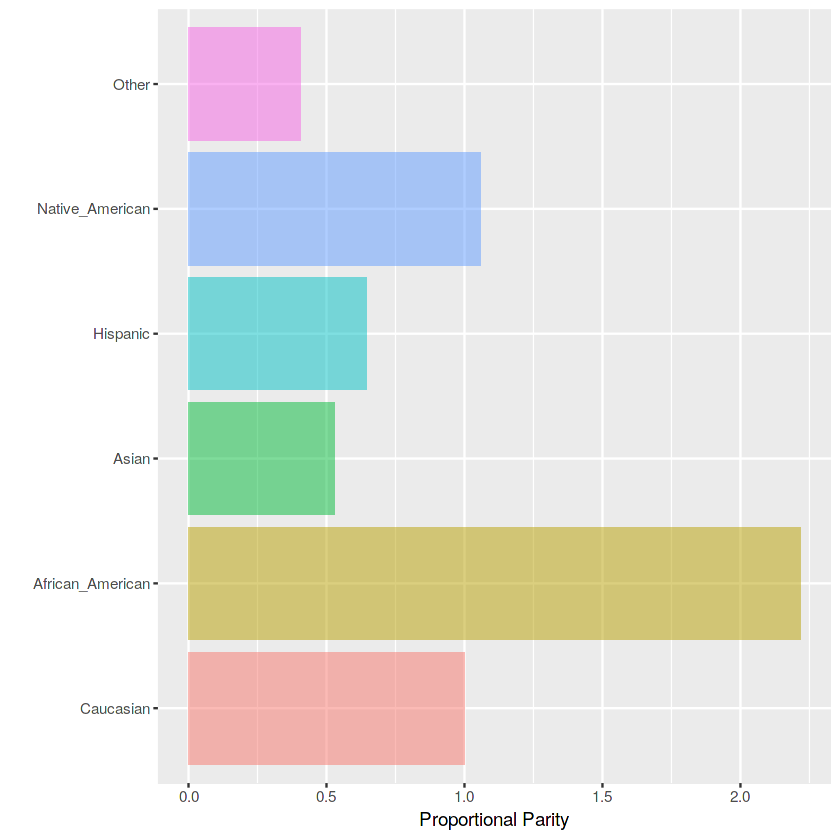The proportional parity still shows that African-American defendants are treated unfairly by our model. At the same time, the disparity is lower compared to the one observed with the demographic parity.

All the remaining fairness metrics account for both model predictions and the true labels.

## Equalized odds

Equalized odds, also known as separation, are achieved if the sensitivities in the subgroups are close to each other. The group-specific sensitivities indicate the number of the true positives divided by the total number of positives in that group.

Formula: TP / (TP + FN)

```res_eq <- equal_odds(data         = df_valid,
outcome      = 'Two_yr_Recidivism_01',
outcome_base = '0',
group        = 'ethnicity',
probs        = 'prob_1',
cutoff       = 0.5,
base         = 'African_American')
res_eq\$Metric
```
African_American Caucasian Asian Hispanic Native_American Other
Sensitivity 0.6996047 0.3659574 0.2500000 0.2600000 0.5000000 0.2142857
Equalized odds 1.0000000 0.5230917 0.3573446 0.3716384 0.7146893 0.3062954
Group size 962 6220 16 144 4 1040

## Accuracy parity

Accuracy parity is achieved if the accuracies (all accurately classified examples divided by the total number of examples) in the subgroups are close to each other.

Formula: (TP + TN) / (TP + FP + TN + FN)

```res_acc <- acc_parity(data    = df_valid,
outcome = 'Two_yr_Recidivism_01',
group   = 'ethnicity',
probs   = 'prob_1',
cutoff  = 0.5,
base    = 'African_American')
res_acc\$Metric
```
African_American Caucasian Asian Hispanic Native_American Other
Accuracy 0.6860707 0.6623794 0.750000 0.6805556 0.750000 0.6730769
Accuracy Parity 1.0000000 0.9654682 1.093182 0.9919613 1.093182 0.9810606
Group size 962 6220 16 144 4 1040

## False negative rate parity

False negative rate parity is achieved if the false negative rates (the ratio between the number of false negatives and the total number of positives) in the subgroups are close to each other.

Formula: FN / (TP + FN)

```res_fnr <- fnr_parity(data         = df_valid,
outcome      = 'Two_yr_Recidivism_01',
outcome_base = '0',
group        = 'ethnicity',
probs        = 'prob_1',
cutoff       = 0.5,
base         = 'African_American')
res_fnr\$Metric
```
African_American Caucasian Asian Hispanic Native_American Other
FNR 0.3003953 0.6340426 0.750000 0.740000 0.500000 0.7857143
FNR Parity 1.0000000 2.1106943 2.496711 2.463421 1.664474 2.6156015
Group size 962 6220 16 144 4 1040

## False positive rate parity

False positive rate parity is achieved if the false positive rates (the ratio between the number of false positives and the total number of negatives) in the subgroups are close to each other.

Formula: FP / (TN + FP)

```res_fpr <- fpr_parity(data         = df_valid,
outcome      = 'Two_yr_Recidivism_01',
outcome_base = '0',
group        = 'ethnicity',
probs        = 'prob_1',
cutoff       = 0.5,
base         = 'African_American')
res_fpr\$Metric
```
African_American Caucasian Asian Hispanic Native_American Other
FPR 0.3289474 0.1576227 0.08333333 0.09574468 0 0.01612903
FPR Parity 1.0000000 0.4791731 0.25333333 0.29106383 0 0.04903226
Group size 962 6220 1600 14400 4 10400

## Negative predictive value parity

Negative predictive value parity is achieved if the negative predictive values in the subgroups are close to each other. The negative predictive value is computed as a ratio between the number of true negatives and the total number of predicted negatives. This function can be considered the ‘inverse’ of the predictive rate parity.

Formula: TN / (TN + FN)

```res_npv <- npv_parity(data         = df_valid,
outcome      = 'Two_yr_Recidivism_01',
outcome_base = '0',
group        = 'ethnicity',
probs        = 'prob_1',
cutoff       = 0.5,
base         = 'African_American')
res_npv\$Metric
```
African_American Caucasian Asian Hispanic Native_American Other
NPV 0.6681223 0.6863158 0.7857143 0.6967213 0.6666667 0.6489362
NPV Parity 1.0000000 1.0272308 1.1760037 1.0428051 0.9978214 0.9712835
Group size 962 6220 160 1440 40 1040

## Specificity parity

Specificity parity is achieved if the specificities (the ratio of the number of the true negatives and the total number of negatives) in the subgroups are close to each other. This function can be considered the ‘inverse’ of the equalized odds.

Formula: TN / (TN + FP)

```res_sp <- spec_parity(data         = df_valid,
outcome      = 'Two_yr_Recidivism_01',
outcome_base = '0',
group        = 'ethnicity',
probs        = 'prob_1',
cutoff       = 0.5,
base         = 'African_American')
res_sp\$Metric
```
African_American Caucasian Asian Hispanic Native_American Other
Specificity 0.6710526 0.8423773 0.9166667 0.9042553 1.000000 0.983871
Specificity Parity 1.0000000 1.2553073 1.3660131 1.3475177 1.490196 1.466161
Group size 962 6220 160 1440 4 104

Apart from the parity-based metrics presented above, two additional comparisons are implemented: ROC AUC comparison and Matthews correlation coefficient comparison.

## ROC AUC parity

This function calculates ROC AUC and visualizes ROC curves for all subgroups. Note that probabilities must be defined for this function. Also, as ROC evaluates all possible cutoffs, the cutoff argument is excluded from this function.

```res_auc <- roc_parity(data         = df_valid,
outcome      = 'Two_yr_Recidivism_01',
group        = 'Female',
probs        = 'prob_1',
base         = 'Male')
res_auc\$Metric
```

Male Female
ROC AUC 0.7221429 0.7192349
ROC AUC Parity 1.0000000 0.9959731
Group size 151 337

Apart from the standard outputs, the function also returns ROC curves for each of the subgroups:

```res_auc\$ROCAUC_plot
```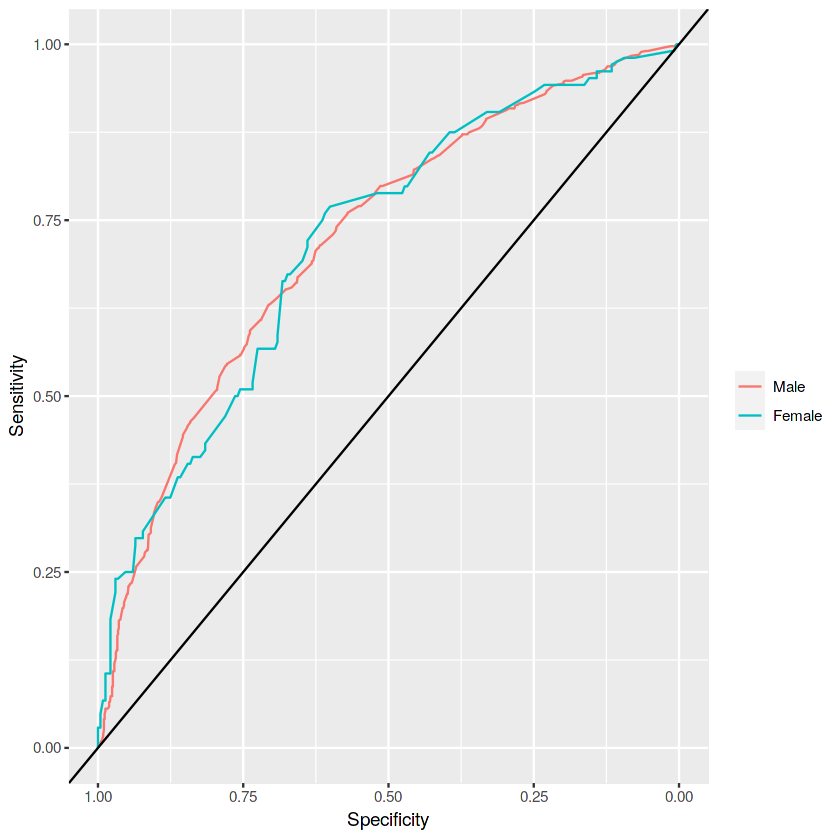## Matthews correlation coefficient parity

The Matthews correlation coefficient (MCC) takes all four classes of the confusion matrix into consideration. MCC is sometimes referred to as the single most powerful metric in binary classification problems, especially for data with class imbalances.

Formula: (TP×TN-FP×FN)/√((TP+FP)×(TP+FN)×(TN+FP)×(TN+FN))

```res_mcc <- mcc_parity(data         = df_valid,
outcome      = 'Two_yr_Recidivism_01',
outcome_base = '0',
group        = 'Female',
probs        = 'prob_1',
cutoff       = 0.5,
base         = 'Male')
res_mcc\$Metric
```
Male Female
MCC 0.3316558 0.2893650
MCC Parity 1.0000000 0.8724859

# 7. Closing words

You have read through the fairness R package tutorial! By now, you should have a solid grip on algorithmic group fairness metrics.

We hope that you will be able to use the R package in your data analysis! Please let me know if you run into any issues while working with the package in the comments below or on GitHub. Please also feel free to contact the authors if you have any feedback.

Acknowlegments:

• Calders, T., & Verwer, S. (2010). Three naive Bayes approaches for discrimination-free classification. Data Mining and Knowledge Discovery, 21(2), 277-292.
• Chouldechova, A. (2017). Fair prediction with disparate impact: A study of bias in recidivism prediction instruments. Big data, 5(2), 153-163.
• Feldman, M., Friedler, S. A., Moeller, J., Scheidegger, C., & Venkatasubramanian, S. (2015, August). Certifying and removing disparate impact. In Proceedings of the 21th ACM SIGKDD International Conference on Knowledge Discovery and Data Mining (pp. 259-268). ACM.
• Friedler, S. A., Scheidegger, C., Venkatasubramanian, S., Choudhary, S., Hamilton, E. P., & Roth, D. (2018). A comparative study of fairness-enhancing interventions in machine learning. arXiv preprint arXiv:1802.04422.
• Zafar, M. B., Valera, I., Gomez Rodriguez, M., & Gummadi, K. P. (2017, April). Fairness beyond disparate treatment & disparate impact: Learning classification without disparate mistreatment. In Proceedings of the 26th International Conference on World Wide Web (pp. 1171-1180). International World Wide Web Conferences Steering Committee.